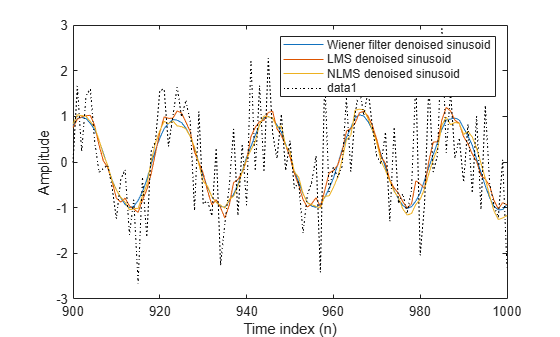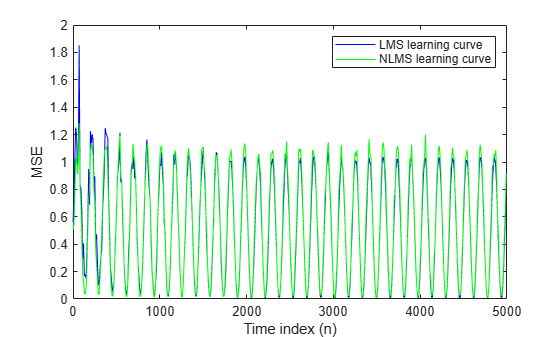# Signal Enhancement Using LMS and NLMS Algorithms

Using the least mean square (LMS) and normalized LMS algorithms, extract the desired signal from a noise-corrupted signal by filtering out the noise. Both of these algorithms are available with the `dsp.LMSFilter `System object™.

### Create the Signals for Adaptation

The desired signal (the output from the process) is a sinusoid with 1000 samples per frame.

`sine = dsp.SineWave('Frequency',375,'SampleRate',8000,'SamplesPerFrame',1000)`
```sine = dsp.SineWave with properties: Amplitude: 1 Frequency: 375 PhaseOffset: 0 ComplexOutput: false Method: 'Trigonometric function' SampleRate: 8000 SamplesPerFrame: 1000 OutputDataType: 'double' ```
`s = sine();`

To perform adaptation, the filter requires two signals:

• A reference signal

• A noisy signal that contains both the desired signal and an added noise component

#### Generate the Noise Signal

Create a noise signal with autoregressive noise (defined as `v1`). In autoregressive noise, the noise at time t depends only on the previous values and a random disturbance.

```v = 0.8*randn(sine.SamplesPerFrame,1); % Random noise part. ar = [1,1/2]; % Autoregression coefficients. ARfilt = dsp.IIRFilter('Numerator',1,'Denominator',ar)```
```ARfilt = dsp.IIRFilter with properties: Structure: 'Direct form II transposed' Numerator: 1 Denominator: [1 0.5000] InitialConditions: 0 Show all properties ```
`v1 = ARfilt(v);`

#### Corrupt the Desired Signal to Create a Noisy Signal

To generate the noisy signal that contains both the desired signal and the noise, add the noise signal `v1` to the desired signal `s`. The noise-corrupted sinusoid `x` is:

`x = s + v1;`

Adaptive filter processing seeks to recover `s` from `x` by removing `v1`. To complete the signals needed to perform adaptive filtering, the adaptation process requires a reference signal.

#### Create a Reference Signal

Define a moving average signal `v2` that is correlated with `v1`. The signal `v2` is the reference signal for this example.

```ma = [1, -0.8, 0.4, -0.2]; MAfilt = dsp.FIRFilter('Numerator',ma)```
```MAfilt = dsp.FIRFilter with properties: Structure: 'Direct form' NumeratorSource: 'Property' Numerator: [1 -0.8000 0.4000 -0.2000] InitialConditions: 0 Show all properties ```
`v2 = MAfilt(v);`

Two similar, sixth-order adaptive filters — LMS and NLMS — form the basis of this example. Set the order as a variable in MATLAB™ and create the filters.

```L = 7; lms = dsp.LMSFilter(L,'Method','LMS')```
```lms = dsp.LMSFilter with properties: Method: 'LMS' Length: 7 StepSizeSource: 'Property' StepSize: 0.1000 LeakageFactor: 1 InitialConditions: 0 AdaptInputPort: false WeightsResetInputPort: false WeightsOutput: 'Last' Show all properties ```
`nlms = dsp.LMSFilter(L,'Method','Normalized LMS')`
```nlms = dsp.LMSFilter with properties: Method: 'Normalized LMS' Length: 7 StepSizeSource: 'Property' StepSize: 0.1000 LeakageFactor: 1 InitialConditions: 0 AdaptInputPort: false WeightsResetInputPort: false WeightsOutput: 'Last' Show all properties ```

### Choose the Step Size

LMS-like algorithms have a step size that determines the amount of correction applied as the filter adapts from one iteration to the next. A step size that is too small increases the time for the filter to converge on a set of coefficients. A step size that is too large might cause the adapting filter to diverge and never reach convergence. In this case, the resulting filter might not be stable.

As a rule of thumb, smaller step sizes improve the accuracy with which the filter converges to match the characteristics of the unknown system, at the expense of the time it takes to adapt.

The `maxstep` function of `dsp.LMSFilter` object determines the maximum step size suitable for each LMS adaptive filter algorithm that ensures that the filter converges to a solution. Often, the notation for the step size is µ.

`[mumaxlms,mumaxmselms] = maxstep(lms,x)`
```mumaxlms = 0.2127 ```
```mumaxmselms = 0.1312 ```
`[mumaxnlms,mumaxmsenlms] = maxstep(nlms,x)`
```mumaxnlms = 2 ```
```mumaxmsenlms = 2 ```

### Set the Adapting Filter Step Size

The first output of the `maxstep` function is the value needed for the mean of the coefficients to converge, while the second output is the value needed for the mean squared coefficients to converge. Choosing a large step size often causes large variations from the convergence values, so generally choose smaller step sizes.

`lms.StepSize = mumaxmselms/30 `
```lms = dsp.LMSFilter with properties: Method: 'LMS' Length: 7 StepSizeSource: 'Property' StepSize: 0.0044 LeakageFactor: 1 InitialConditions: 0 AdaptInputPort: false WeightsResetInputPort: false WeightsOutput: 'Last' Show all properties ```
`nlms.StepSize = mumaxmsenlms/20 `
```nlms = dsp.LMSFilter with properties: Method: 'Normalized LMS' Length: 7 StepSizeSource: 'Property' StepSize: 0.1000 LeakageFactor: 1 InitialConditions: 0 AdaptInputPort: false WeightsResetInputPort: false WeightsOutput: 'Last' Show all properties ```

### Filter with the Adaptive Filters

You have set up the parameters of the adaptive filters and are now ready to filter the noisy signal. The reference signal v2 is the input to the adaptive filters. x is the desired signal in this configuration.

Through adaptation, y, the output of the filters, tries to emulate x as closely as possible.

Since v2 is correlated only with the noise component v1 of x, it can only really emulate v1. The error signal (the desired x), minus the actual output y, constitutes an estimate of the part of x that is not correlated with v2 — s, the signal to extract from x.

```[~,elms,wlms] = lms(v2,x); [~,enlms,wnlms] = nlms(v2,x);```

### Compute the Optimal Solution

For comparison, compute the optimal FIR Wiener filter.

```reset(MAfilt); bw = firwiener(L-1,v2,x); % Optimal FIR Wiener filter MAfilt = dsp.FIRFilter('Numerator',bw)```
```MAfilt = dsp.FIRFilter with properties: Structure: 'Direct form' NumeratorSource: 'Property' Numerator: [1.0001 0.3060 0.1050 0.0482 0.1360 0.0959 0.0477] InitialConditions: 0 Show all properties ```
```yw = MAfilt(v2); % Estimate of x using Wiener filter ew = x - yw; % Estimate of actual sinusoid```

### Plot the Results

Plot the resulting denoised sinusoid for each filter — the Wiener filter, the LMS adaptive filter, and the NLMS adaptive filter — to compare the performance of the various techniques.

```n = (1:1000)'; plot(n(900:end),[ew(900:end), elms(900:end),enlms(900:end)]) legend('Wiener filter denoised sinusoid',... 'LMS denoised sinusoid','NLMS denoised sinusoid') xlabel('Time index (n)') ylabel('Amplitude')```

As a reference point, include the noisy signal as a dotted line in the plot.

```hold on plot(n(900:end),x(900:end),'k:') xlabel('Time index (n)') ylabel('Amplitude') hold off```### Compare the Final Coefficients

Finally, compare the Wiener filter coefficients with the coefficients of the adaptive filters. While adapting, the adaptive filters try to converge to the Wiener coefficients.

`[bw.' wlms wnlms]`
```ans = 7×3 1.0001 0.8644 0.9690 0.3060 0.1198 0.2661 0.1050 -0.0020 0.1226 0.0482 -0.0046 0.1074 0.1360 0.0680 0.2210 0.0959 0.0214 0.1940 0.0477 0.0292 0.1127 ```

### Reset the Filter Before Filtering

You can reset the internal filter states at any time by calling the `reset` function on the filter object.

For instance, these successive calls produce the same output after resetting the object.

```[ylms,elms,wlms] = lms(v2,x); [ynlms,enlms,wnlms] = nlms(v2,x);```

If you do not reset the filter object, the filter uses the final states and coefficients from the previous run as the initial conditions and data set for the next run.

### Investigate Convergence Through Learning Curves

To analyze the convergence of the adaptive filters, use the learning curves. The toolbox provides methods to generate the learning curves, but you need more than one iteration of the experiment to obtain significant results.

This demonstration uses 25 sample realizations of the noisy sinusoids.

```reset(ARfilt) reset(sine); release(sine); n = (1:5000)'; sine.SamplesPerFrame = 5000```
```sine = dsp.SineWave with properties: Amplitude: 1 Frequency: 375 PhaseOffset: 0 ComplexOutput: false Method: 'Trigonometric function' SampleRate: 8000 SamplesPerFrame: 5000 OutputDataType: 'double' ```
```s = sine(); nr = 25; v = 0.8*randn(sine.SamplesPerFrame,nr); ARfilt = dsp.IIRFilter('Numerator',1,'Denominator',ar)```
```ARfilt = dsp.IIRFilter with properties: Structure: 'Direct form II transposed' Numerator: 1 Denominator: [1 0.5000] InitialConditions: 0 Show all properties ```
```v1 = ARfilt(v); x = repmat(s,1,nr) + v1; reset(MAfilt); MAfilt = dsp.FIRFilter('Numerator',ma)```
```MAfilt = dsp.FIRFilter with properties: Structure: 'Direct form' NumeratorSource: 'Property' Numerator: [1 -0.8000 0.4000 -0.2000] InitialConditions: 0 Show all properties ```
`v2 = MAfilt(v);`

### Compute the Learning Curves

Now compute mean squared error. To speed things up, compute the error every 10 samples.

First, reset the adaptive filters to avoid using the coefficients it has already computed and the states it has stored. Then plot the learning curves for the LMS and NLMS adaptive filters.

```reset(lms); reset(nlms); M = 10; % Decimation factor mselms = msesim(lms,v2,x,M); msenlms = msesim(nlms,v2,x,M); plot(1:M:n(end),mselms,'b',1:M:n(end),msenlms,'g') legend('LMS learning curve','NLMS learning curve') xlabel('Time index (n)') ylabel('MSE')```In this plot you see the calculated learning curves for the LMS and NLMS adaptive filters.

### Compute the Theoretical Learning Curves

For the LMS and NLMS algorithms, functions in the toolbox help you compute the theoretical learning curves, along with the minimum mean squared error (MMSE), the excess mean squared error (EMSE), and the mean value of the coefficients.

MATLAB might take some time to calculate the curves. The figure shown after the code plots the predicted and actual LMS curves.

```reset(lms); [mmselms,emselms,meanwlms,pmselms] = msepred(lms,v2,x,M); x = 1:M:n(end); y1 = mmselms*ones(500,1); y2 = emselms*ones(500,1); y3 = pmselms; y4 = mselms; plot(x,y1,'m',x,y2,'b',x,y3,'k',x,y4,'g') legend('MMSE','EMSE','Predicted LMS learning curve',... 'LMS learning curve') xlabel('Time index (n)') ylabel('MSE')```Watch now